.NET Framework 4.5.2+

# IWorkbook.Worksheets Property

Returns the collection of worksheets in a workbook.

## Declaration

``WorksheetCollection Worksheets { get; }``

## Property Value

Type Description
WorksheetCollection

The collection of Worksheet objects.

## Remarks

Use the WorksheetCollection object’s members to access, add, and remove worksheets.

### Access a Worksheet

Use the WorksheetCollection.Item property to access a worksheet in a workbook.

#### Obtain the worksheet at the specified index

``````using DevExpress.Spreadsheet;
// ...

// Access the worksheet collection.
WorksheetCollection worksheets = workbook.Worksheets;

// Access the first worksheet.
Worksheet worksheet1 = workbook.Worksheets;
``````

A worksheet index is zero-based. It specifies the worksheet position within a collection.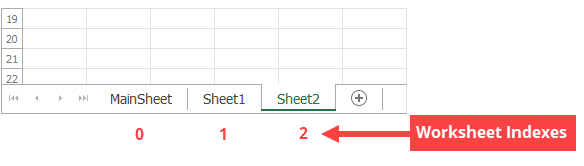#### Obtain the worksheet with a given name

``````using DevExpress.Spreadsheet;
// ...

//  Access the worksheet collection.
WorksheetCollection worksheets = workbook.Worksheets;

// Access the worksheet with the specified name.
Worksheet worksheet2 = workbook.Worksheets["MainSheet"];
``````

A worksheet name is unique within the collection and is shown on a worksheet tab.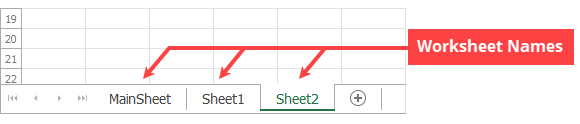### Set an Active Worksheet

Use the WorksheetCollection.ActiveWorksheet property to specify the active worksheet in a workbook.

``````using DevExpress.Spreadsheet;
// ...

// Set "Sheet2" as the active worksheet.
workbook.Worksheets.ActiveWorksheet = workbook.Worksheets["Sheet2"];
``````

Use the WorksheetCollection.Add method to add a worksheet to the end of the worksheet collection.

``````using DevExpress.Spreadsheet;
// ...

// Add a worksheet with the default name.
// Default names are "Sheet1", "Sheet2", ..., "SheetN".

// Add new worksheets with the specified names.

``````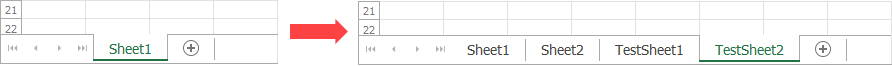The WorksheetCollection.Insert method allows you to insert a worksheet at the specified position in the collection.

``````using DevExpress.Spreadsheet;
// ...

// Insert a worksheet at the second position in the workbook.
workbook.Worksheets.Insert(1, "TestSheet3");

// Insert a worksheet with the default name at the fourth position in the workbook.
workbook.Worksheets.Insert(3);
``````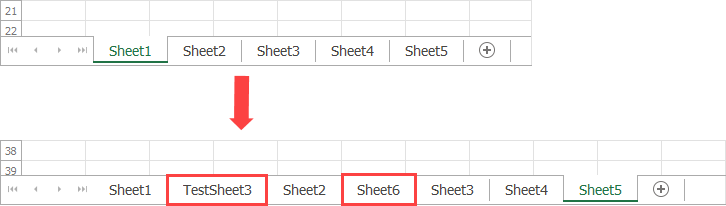### Delete a Worksheet

Use the following methods to delete a worksheet:

Note

A workbook must contain at least one visible worksheet.

``````using DevExpress.Spreadsheet;
// ...

// Delete the first worksheet from the workbook.
workbook.Worksheets.RemoveAt(0);

// Delete the "Sheet2" worksheet from the workbook.
workbook.Worksheets.Remove(workbook.Worksheets["Sheet2"]);
``````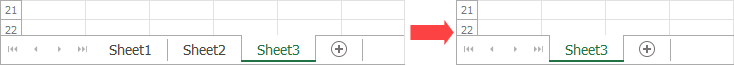The following code snippets (auto-collected from DevExpress Examples) contain references to the Worksheets property.

Note

The algorithm used to collect these code examples remains a work in progress. Accordingly, the links and snippets below may produce inaccurate results. If you encounter an issue with code examples below, please use the feedback form on this page to report the issue.